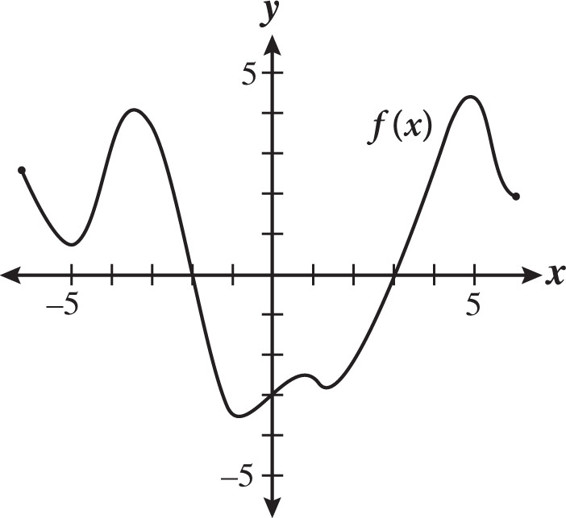# SAT Math Multiple Choice Question 304: Answer and Explanation

### Test Information

Question: 304

4.The figure above shows the graph of f(x). For which value(s) of x does f(x) equal 0?

• A. 3 only
• B. -3 only
• C. -2 and 3
• D. -3, -2, and 3